/

### Function Description

Returns an array corresponding to the AR(1) weighted de-smoothed (or ‘de-correlated’) values of a series, as described in the book Extreme Events. This involves:

(a)    Postulating (for, say, a return series) that there is some underlying ‘true’ return series,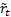, and that the observed series,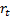, derives from it via a first order autoregressive model,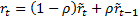;

(b)   Identifying the value of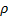(between 0 and 0.5) for whichhas zero weighted first order correlation (which implies that the weighted covariance between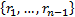and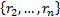is zero; and

(c)    Using this value ofderivingusing the following formula: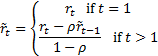In step (b) we weight the elements in the covariance computation using weights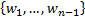(where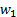corresponds to the weight given to the component arising from the term in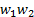.

The value ofin (b) can be accessed using MnWeightedDesmooth_AR1_rho.

If the weights are equal then returns the same as MnDesmooth_AR1.

Contents | Prev | Next